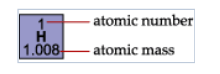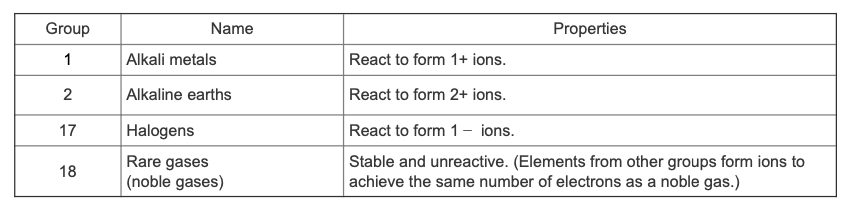Problem: Part B.  If a new element were discovered and it was found to form 1− ions, in which group would you place it in the periodic table? Enter the group number.The periodic table is a list of elements arranged by atomic number. As you can see in the image below, the atomic number for each element is the whole number in the cell. The atomic mass, written below the symbol here although it may appear elsewhere in some periodic tables, is the average mass of the isotopes of that element.Groups and periodsColumns in the periodic table are called groups, and the rows are called periods. Notice that the groups are labeled 1 to 18 and the periods are labeled 1 to 7. Groups 1, 2, and 13 to 18 are called the main group elements, groups 3 to 12 are the transition elements, and the bottom two rows are called the lanthanides and actinides, respectively.This table gives properties and alternate names for some of the groups:Metals vs. nonmetalsNotice the heavy zigzag line running diagonally across the right part of the table. This line separates metals (below and to the left of the line) from the nonmetals (above and to the right of the line). Metals tend to lose electrons to form positive ions, whereas nonmetals tend to gain electrons to form negative ions.

FREE Expert Solution
97% (146 ratings)
FREE Expert Solution

We are being asked to determine the group of the new element that form -1 ions.

Recall that when becoming an ion, the element tries to achieve the configuration of the nearest noble gas (Group 8A or Group 18). For each group in the periodic table, we have:

97% (146 ratings)
Problem Details

Part B.  If a new element were discovered and it was found to form 1− ions, in which group would you place it in the periodic table?

Enter the group number.

The periodic table is a list of elements arranged by atomic number. As you can see in the image below, the atomic number for each element is the whole number in the cell. The atomic mass, written below the symbol here although it may appear elsewhere in some periodic tables, is the average mass of the isotopes of that element.Groups and periods

Columns in the periodic table are called groups, and the rows are called periods. Notice that the groups are labeled 1 to 18 and the periods are labeled 1 to 7. Groups 1, 2, and 13 to 18 are called the main group elements, groups 3 to 12 are the transition elements, and the bottom two rows are called the lanthanides and actinides, respectively.

This table gives properties and alternate names for some of the groups:Metals vs. nonmetals

Notice the heavy zigzag line running diagonally across the right part of the table. This line separates metals (below and to the left of the line) from the nonmetals (above and to the right of the line). Metals tend to lose electrons to form positive ions, whereas nonmetals tend to gain electrons to form negative ions.